• 2,\cdots,k_s k1​,k2​,⋯,ks​,使 k 1 α 1 + k 2 α 2 + ⋯ + k s α s = 0 k_1\alpha_1+k_2\alpha_2+\cdots+k_s\alpha_s=0 k1​α1​+k2​α2​+⋯+ks​αs​=0 例题： 判断下列向量组是否线性相关： α 1 = [ ...
定义： 向量组

α

1

,

α

2

,

⋯

,

α

s

(

s

⩾

1

)

\alpha_1,\alpha_2,\cdots,\alpha_s(s\geqslant1)

称为线性相关,如果有数域

P

P

中不全为零的数

k

1

,

k

2

,

⋯

,

k

s

k_1,k_2,\cdots,k_s

,使

k

1

α

1

+

k

2

α

2

+

⋯

+

k

s

α

s

=

0

k_1\alpha_1+k_2\alpha_2+\cdots+k_s\alpha_s=0

例题： 判断下列向量组是否线性相关：

α

1

=

[

−

2

−

5

−

3

−

4

]

,

α

2

=

[

−

5

11

3

10

]

,

α

3

=

[

−

3

−

7

−

1

−

6

]

,

α

4

=

[

−

13

−

30

−

1

2

−

26

]

,

\begin{aligned} \alpha_1=\begin{bmatrix}\phantom{-}2\\-5\\\phantom{-}3\\-4\end{bmatrix}, \alpha_2=\begin{bmatrix}-5\\11\\3\\10\end{bmatrix}, \alpha_3=\begin{bmatrix}-3\\\phantom{-}7\\-1\\\phantom{-}6\end{bmatrix}, \alpha_4=\begin{bmatrix}\phantom{-}13\\-30\\\phantom{-1}2\\-26\end{bmatrix}, \end{aligned}

例题来源：《高等代数学习指导书》.丘维声著.第二版.P76

解法一：定义法：
设

k

1

,

k

2

,

k

3

,

k

4

∈

R

k_1,k_2,k_3,k_4\in R

满足

k

1

α

1

+

k

2

α

2

+

k

3

α

3

+

k

4

α

4

=

0

k_1\alpha_1+k_2\alpha_2+k_3\alpha_3+k_4\alpha_4=0

即：

{

2

k

1

−

5

k

2

−

3

k

3

+

13

k

4

=

0

−

5

k

1

+

11

k

2

7

k

3

−

30

k

4

=

0

3

k

1

+

3

k

2

−

1

k

3

+

2

k

4

=

0

−

4

k

1

+

10

k

2

6

k

3

−

26

k

4

=

0

\left\{\begin{aligned} 2k_1&-5k_2&-3k_3&+13k_4&=0\\ -5k_1&+11k_2&7k_3&-30k_4&=0\\ 3k_1&+3k_2&-1k_3&+2k_4&=0\\ -4k_1&+10k_2&6k_3&-26k_4&=0\\ \end{aligned}\right.

写成矩阵形式即为：

[

2

−

5

−

3

13

−

5

11

7

−

30

3

3

−

1

2

−

4

10

6

−

26

]

[

k

1

k

2

k

3

k

4

]

=

[

0

0

0

0

]

\begin{bmatrix} 2&-5&-3&13\\ -5&11&7&-30\\ 3&3&-1&2\\ -4&10&6&-26\\ \end{bmatrix}\begin{bmatrix}k_1\\k_2\\k_3\\k_4\end{bmatrix}=\begin{bmatrix}0\\0\\0\\0\end{bmatrix}

经过矩阵的初等行变换，我们得到上面的方程组与下面的方程组等价：

[

1

0

−

2

3

7

3

0

1

1

3

−

5

3

0

0

0

0

0

0

0

0

]

[

k

1

k

2

k

3

k

4

]

=

[

0

0

0

0

]

\begin{bmatrix} 1&0&-\frac{2}{3}&\frac{7}{3}\\ 0&1&\frac{1}{3}&-\frac{5}{3}\\ 0&0&0&0\\ 0&0&0&0\\ \end{bmatrix}\begin{bmatrix}k_1\\k_2\\k_3\\k_4\end{bmatrix}=\begin{bmatrix}0\\0\\0\\0\end{bmatrix}

显然,系数矩阵的行列式等于零,从而方程有非零解。所以向量组线性相关

如果题目只问向量组是否相关，可以只作答到这一步。下面的步骤是为了求出一组不为零的系数k

方程的一般解为

{

x

1

=

2

3

x

3

−

7

3

x

4

x

2

=

−

1

3

x

3

+

5

3

x

4

\left\{\begin{aligned} x_1&=\frac{2}{3}x_3-\frac{7}{3}x_4\\ x_2&=-\frac13x_3+\frac53x_4 \end{aligned}\right.

其中一个特解为

k

1

=

3

,

k

2

=

−

2

,

k

3

=

1

,

k

4

=

−

1

k_1=3,k_2=-2,k_3=1,k_4=-1

从而：

3

α

1

−

2

α

2

+

α

3

−

α

4

=

0

3\alpha_1-2\alpha_2+\alpha_3-\alpha_4=0

总结：

设

k

1

α

1

+

k

2

α

2

+

⋯

+

k

n

α

n

=

0

k_1\alpha_1+k_2\alpha_2+\cdots+k_n\alpha_n=0

把 1. 中的方程写成矩阵的形式，化成阶梯型行列式，即可判断是否存在非零解求出一组非零解

2021年1月4日19:26:01

如有问题请在评论区留言

2021年6月26日 有改动
展开全文• 数据结构-线性相关代码
1.汉诺塔-递归问题

int i=1;//记录步数
void move(int n,char from,char to) {
printf("第%d步:将%d号盘子%c---->%c\n",i++,n,from,to);
}
void hanoi(int n,char from,char denpend_on,char to) {
if (n==1)
move(1,from,to);//只有一个盘子是直接将初塔上的盘子移动到目的地
else {
hanoi(n-1,from,to,denpend_on);
move(n,from,to);
hanoi(n-1,denpend_on,from,to);
}


2.已知一个不带表头节点的单链表中每个节点存放一个整数，并且节点数不少于2。设计一个算法，判断该链表中从第2个节点开始（包含第2个节点）的每个元素值是否等于其序号的平方减去其前驱结点的值，满足则返回true，否则返回false。

bool judge(LinkList head){
int num=2;
while(p){
if(p->data!=num*num*q->data)
return false;
q=p;
p=p->next;
}
return true;
}


3.假定数组A[0,…,n-1]中有多个零元素，试写一个函数，将A中所有的非零元素依次移到数组A的前端，要求空间复杂度为O(1)。

void MoveNumZeroLeft(int A[],int n){
int i=0,j=n-1;
while(i<j){
while(A[i]!=0&&i<j)	i++;
while(A[j]==0&&i<j) j--;
if(i<j)	Swap(A[i],A[j]);
}
}


LNode * FindKthToTail(LinkList L,int k){
LNode *q=NULL;
for(int i=0;i<k-1;i++)
p=p->next;
while(q->next){
p=p->next;
q=q->next;
}
return q;
}


5.将n(n>1)个整数存放到一维数组R中，试设计一个在时间和空间上尽可能高效的算法，将R中保存的序列循环左移p(0<p<n)个位置，即将R中的数据由(X0,X1,…,Xn-1)变换成为(Xp,Xp+1,…,X0,X1,…,Xp-1)。

void Converse(int R[],int n,int p){	//R为数组，n为长度，p为要左循环多少个
Reverse(R,0,p-1);
Reverse(R,p,n-1);
Reverse(R,0,n-1);
}
void Reverse(int R[],int from,int to){
for(int i=0;i<(to-from+1)/2;i++)
Swap(R[from+i],R[to-i]);
}


6.编写一个算法，将一个非负的十进制数N转换为一个二进制数。

void DecToBin(int N){
int i;
Stack S;
while(N!=0){
i=N%2;
N=N/2;
Push(S,i);
}
while(IsEmpty(S))
printf(“%d”,Pop(S));
}



展开全文数据结构 递归
• ## 线性判别函数

千次阅读 2015-12-01 16:10:57
最近看到线性判别分析LDA，然后发现网上有少量的文章说线性判别函数和LDA很相似，当时就感觉虽然名字很像，但这完全是两个不相关的模型。所以就把这些东西详细地区别了一下。 一、线性判别函数 1、函数是对模式进行...
最近看到线性判别分析LDA，然后发现网上有少量的文章说线性判别函数和LDA很相似，当时就感觉虽然名字很像，但这完全是两个不相关的模型。所以就把这些东西详细地区别了一下。
一、线性判别函数
1、函数是对模式进行分类的准则函数，因此非常明显线性判别函数是用来进行模式识别的。若分属于ω1，ω2的两类模式可用一方程d(X) =0来划分，那么称d(X) 为判别函数，或称判决函数、决策函数。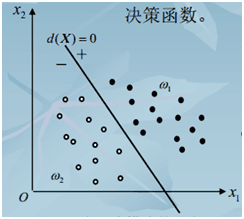例：一个二维的两类判别问题，模式分布如图示，这些分属于ω1，ω2两类的模式可用一直线方程d(X)=0来划分。d( X) = w1 x1 + w2 x2 +w3 = 0，式中x1 , x2 为坐标变量，w1 , w2, w3 为方程参数。
将某一未知模式 X代入：d( X) ，若d( X)>0，则属于ω1类，若d( X)<0，则属于ω2类。若d( X)=0，则可以考虑拒绝或者做出其他判断。判别函数d(X)可以是线性的或者非线性的函数，我们只讨论线性的，对于非线性的问题在广义线性判别函数的时候会将所有非线性的问题转换为线性问题。
在上面的例子中，我们讨论的是在一个二维的平面内进行分类，其实在大多时候数据的维度都远远的要超过二维。特别是在线性函数无法分割数据的时候往往需要把数据扩展到更高维度，才能用线性函数进行分割。
d( X) = w1 x1 + w2 x2 + …+wn xn+w0，X=[x1,x2,…,xn,1]，参数向量W=[w1,w2,…,wn,w0]
当有两类的时候用一个判别函数d1(X)即可实现分类，但是对于多类的情况，有三种划分方式：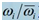两分法，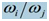两分法，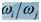两分法特例。
2、两法用线性判别函数将属于ωi类的模式与其余不属于ωi类的模式分开此法将 M个多类问题分成M个两类问题，识别每一类均需M个判别函数。识别出所有的M类仍是这M个函数。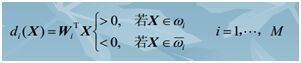（1.1式）
考虑所有的情况，发现上式中并没有考虑所有的情况，比如没有考虑到所有的判别式都为小于零的情况，和多个判别函数同时大于零的情况。下图考虑了分为三类的时候，可能出现的情况。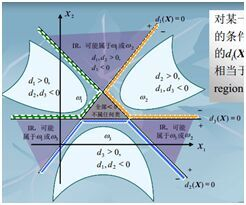比如当有M类的时候，如果每条判别函数相互不平行而且交点至今没有重合，X所在的n维空间会被分成（m+1）m/2+1个区域，而只有m个区域里面的点才能满足1.1式，而剩下的（m+1）m/2+1-m个区域都无法进行明确的分类。这也是目前存在的问题。
3、两分法，一个判别界面只能分开两类，不能把其余所有的类都分开，判决函数为dij( X)=WijX，这里dij=-dji。判别函数的性质dij(X)>0,对所有的j=1…m，若x属于第i类。这种方法一共需要（m+1）m/2个判别式。
4、两分法特例，当ωi /ωj两分法中的判别函数dij(X) ，可以定义为dij ( X) = di (X)- d j(X)时，那么di(X)>dj(X)就相当于多类情况2中的dij(X) >0。此时有m个判别函数di(X)=WiX。判别函数的性质为：di (X)=max{dk(X) k=1…m},若X属于第i类。可以通过dij ( X) = di (X)- d j(X)发现，这种分类方法只不过是第二种分类方法的特例，如果这种分类方法可分则第二种分类方法必定可分，如果第二种分类方法可分，这种方法未必可分。
二、广义线性判别函数
在上面曾经提到低维空间的非线性问题可以在高维空间变成线性问题。上面讲的所有都是在X所在的空间中线性可分为前提条件，是因为即使原始数据D在原来空间线性不可分，我们只需要把低维空间的D扩展到高维空间的X就线性可分了。下面举两个例子：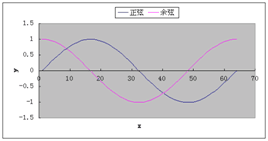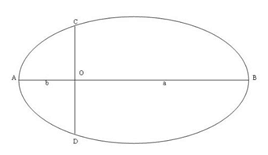比如在二维平面内需要一个正玄曲线y-sin(X)=0才能将数据准确的分割为两类。此时数据D=(x,y),如果把数据扩展到更高维度的空间T，T=(x,y,sin(x),sin(y),1)，那么对于T空间的数据肯定可以找到w1x+w1y+w3(sin(X))+w4(sin(y))+w0=0这样一个线性表达式来使得数据可以划分。针对在二维空间需要椭圆曲线才能划分的数据只需要将D=(x,y)扩展到 T=(x,y,x^2,y^2,xy,1)空间，则在T所在的空间就可以将数据线性划分了。实际上在建模的时候首先要选定的就是要把原始的数据D怎样扩展到更高维度的空间，数据才能变得线性可分。这是建立模型的关键步骤。
三、判别式的求取方法
在上面介绍了模型，也就是建模的时候要做哪些工作。下面的工作就是解模了，这里介绍了三种求取判别式的方法。分别为感知器算法、梯度法、最小误差平方法。接下来介绍的三种方法都是以两类为例介绍的。
1、感知器算法
感知器算法算是一种最简单的算法了，基本上就是一种错了就改的思想，就像是教育小孩子对了的话就告诉他对了不用改正，错了的话就要向正确的方向改正一样。两类线性可分的模式类：w1，w2 ，线性判别函数d(X),若d(X)>0，则X属于w1，若d(X)<0，则X属于w2.首先将数据增加一个维度，所有数据在此维度上的值都是1。然后将属于w2类的数据全部乘上-1，这样处理后的样本记为X’，那么对于X’中的任意一组数据都应该满足d(X’)>0。感知器算法的步骤为：
第一步：选择N个分属于ω1和 ω2类的模式样本构成训练样本集{X1, …, XN}进行增广（扩展一个维度，所有数据在此维度上的值都为1）和规范化处理（上面所说的对于d(X)<0的情况，数据乘以-1的操作）。任取权向量初始值W(1)，开始迭代。迭代次数k=1 。
第二步：用全部训练样本进行一轮迭代，计算W (k)Xi的值，并修正权向量。分两种情况，更新权向量的值：若W (k)Xi≤ 0，分类器对第i个模式做了错误分类，权向量校正为：W(k +1) = W(k) + cXi，c为矫正增量为正值，这个值需要自己设定。若W (k)Xi> 0,表示分类正确则不需要改变权向量:W(k +1) = W(k)
统一写为：若W (k) Xi> 0，分类正确，权向量不变：W(k +1) = W(k)
W (k) Xi <= 0,分类错误，权向量改变：W(k +1) =W(k) + cXi
第三步：分析分类结果：只要有一个错误分类，回到第二步，直至对所有样本正确分类。
对于为什么在判断错的时候要做W(k +1)=W(k) + cXi这样的改变，W(k +1) Xi= W(k) Xi+c Xi^2,所以W(k +1) Xi> W(k)Xi，代表k+1次的权值在对Xi数据的分类情况有了改善。可以证明算法在模式类别线性可分的时候是收敛的。对于多类的问题感知器算法的基本思想还是不变的：错了就向好的方向改变，正确就不再改变。
2、梯度法
梯度法一般的规则就是定义损失函数，然后沿着梯度下降使得损失函数下降到极小值点。损失函数定义为J=0.5sum(|WXi|- WXi),当第一眼看到这个损失函数的时候会感到很诧异，因为里面有取绝对值的运算，这种运算是不可导的。下面的Xi代表第i组数据，Xik代表第i组数据的第k维，k=1…n。首先看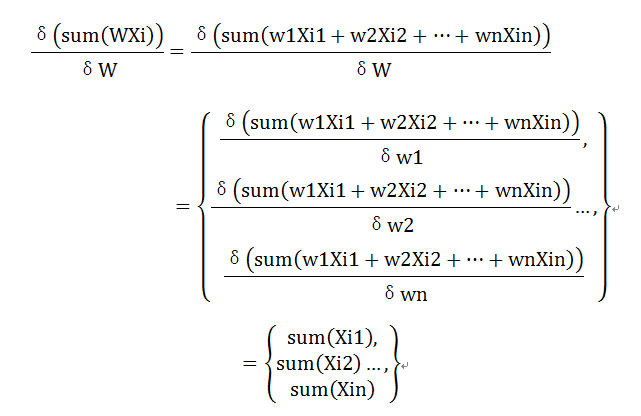同理引入Ti表示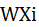的符号，则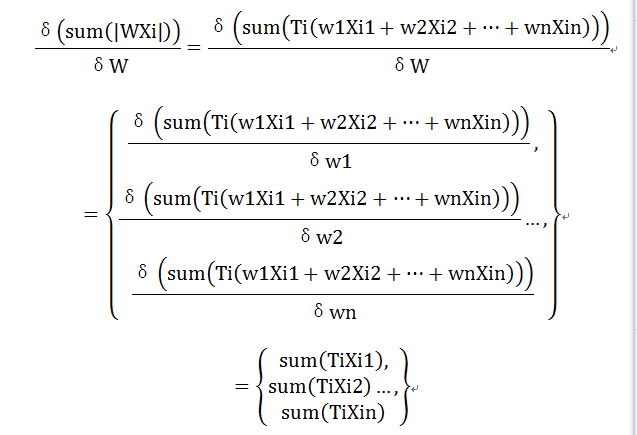所以最后的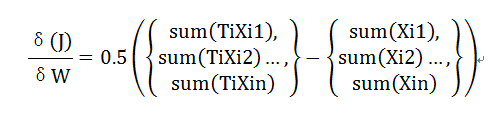权值改变公式为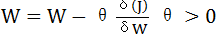，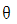为步长，就像感知器算法中的c。只需要如此不断的改变权值，直到权值无法改变即求得了结果。其实通过对比了前面的感知器算法和梯度法会发现，感知器算法每次读入一组数据就改变一次权值，而上面讲的梯度法是所有数据都输入之后，求得所有的 WXi才改变一次权值。严格地说，感知器算法也是梯度法的一种。梯度下降算法改变权值都是通过统一的公式进行调整的，不过我们往往可以控制的是多少组数据改变一次权值，有一组数据改变一次权值的，有所有数据全部输入改变一次权值的，还有介于两者之间的K组数据改变一次权值的。如果要详细了解权值的改变方式可以了解BP神经网络中权值具体改变的方法。不过一般都是设置为输入K组数据改变一次权值，因为像感知器算法那样，每一组数据都改变一次权值，损失函数不是严格梯度下降的，容易导致损失函数震荡。而像上面讲的那样把所有数据输入，求得所有的 WXi才改变一次权值，虽然这样在足够小的理想前提条件下，损失函数会严格的下降，但是当训练数据量很大的时候会造成权值改变速度过慢。所以一般采取折中的方式选择输入K组数据改变一次权值，在机器学习中batchsize一般表示K。
3、最小误差平方法
当把这个方法写出来的时候，感觉这样写好不准确，因为这种方法其实还是梯度下降法，回想当分成两类的时候，要求的这样的一个W，使得满足WXi>0,i=1…，也就是对所有的Xi都要满足WXi>0。既然我们要满足这么一个条件，那么WXi=C>0,是不是也恒定满足这样的条件呢，C代表随便一个正的常数。比如C=1的时候，只需要求WXi=1的解即可，求得W必定满足WXi>0，因为WXi=1。所有此时转换为对方程组的求解了，但是实际问题并不像我们想的那样简单，为什么？因为我们求解的参数个数可能远小于方程组的个数，很有可能根本没有解，因为样本的数量远大于样本的维度再正常不过了。所以还是通过定义损失函数来进行求解最好不过，定义损失函数为J=0.5sum((WXi-1)^2),这时候有人会想C为什么要等于1，而不是等于其他常数，其实很简单，C等于任意一个正整数求得的结果都是一样的，WXi=1求得W记为W(1)，WXi=2求得W记为W(2)，易知W(2)=2 W(1)，所以C无论选取为哪一个正的常数都无所谓，求得的结果都会满足WXi>0，而权向量W在方向上是不会变化的。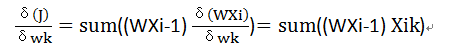然后按照不断的迭代改变权值即可。
四、线性判别函数和SVM
通过看机器学习导论一书可以发现，线性判别函数和支撑向量机SVM放在了同一章节进行讲解了，可以发现线性判别函数和支撑向量机SVM是有很多相似的地方的。基本上都离不开线性这个地方，因为支撑向量机一直解决的问题就是在一个线性可分的空间，如何求出一个超平面将数据最好的分开，注意最好这两个字，而线性判别函数所做的是在一个线性可分的空间，如何求出一个超平面将数据分开。SVM比线性判别函数多了最好两个字，因而就相比之下比线性判别函数的求解复杂了好多，看了那么多关于SVM的资料，感觉最好的就是coursera上面台大林轩田老师开设的机器学习课程。这两个模型基本的思想都是在一个低维空间线性不可分的时候，先把低维空间扩展到高维空间，线性判别函数是是找一个分类超平面，而SVM是找一个最好的分类超平面。
展开全文• 学过线性代数都知道，先求出秩，根据秩的大小与向量的阶数比较判断线性是否相关。求秩matlab用rank函数
学过线性代数都知道，先求出秩，根据秩的大小与向量的阶数比较判断出线性是否相关。求秩matlab用rank函数
rank函数格式
rank(A)

求下列矩阵判断是否线性相关并求出极大无关组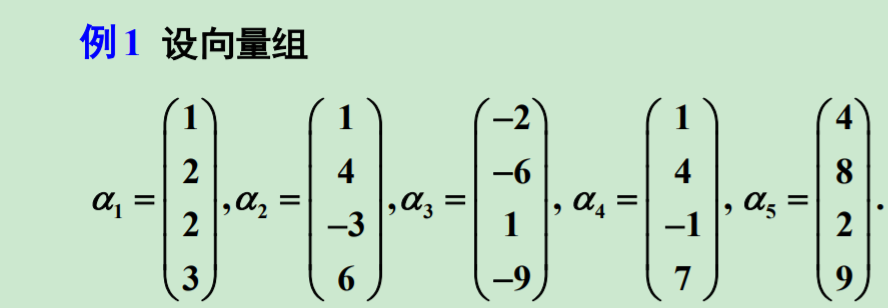>> a1=[1 2 2 3]';
>> a2=[1 4 -3 6]';
>> a3=[-2 -6 1 -9]';
>> a4 = [1 4 -1 7]';
>> a5 = [4 8 2 9]';
>> A=[a1,a2,a3,a4,a5];
>> r=rank(A)

r =

3

>>

然后根据线性代数学科中极大无关组定义将阶梯形矩阵求出来，从右边往做取就行了。 化为阶梯形矩阵
[R,j]=rref(A)
A是矩阵
R是简化后的阶梯形
j是主元

>> [R,j]=rref(A)

R =

1     0    -1     0     4
0     1    -1     0     3
0     0     0     1    -3
0     0     0     0     0

j =

1     2     4

>> A1=A(:,j)

A1 =

1     1     1
2     4     4
2    -3    -1
3     6     7

>>

然后从这里就会发现A1就是它的极大线性无关组。
展开全文matlab 数学建模
• 回归分析-线性相关强度 什么是回归分析呢？ 回归分析：通过建立模型来研究变量之间相互关系的密切程度、结构状态及进行模型预测的一种有效工具 这里，给出回归分析的结构图： 可以看出回归分析的内容实际上非常多。...机器学习
• 线性相关分析是用相关系数来表示两个变量间相互的线性关系，并判断其密切程度的统计方法。 为了下面编程方便起见，我们令X=身高，Y=体重。 > x=ug$height > y=ug$weight > par(mfrow=c(1,2)) > plot...
• 在很多机器学习算法中，都会遇到最优化问题。因为我们机器学习算法，就是要在模型空间中...很多最优化问题都是在目标函数是凸函数或者凹函数的基础上进行的。原因很简单，凸函数的局部极小值就是其全局最小值，凹函数的机器学习 优化
• 在了解线性函数前，先来了解一下线性回归和逻辑回归的区别。线性回归为估值而生，逻辑回归而分类而生。比如我们根据根据一家企业的运营质量、盈利、运营时间等来对企业进行估值，这属于估值问题，如果存在线性关系，...python 机器学习 数据挖掘
• 1.1 皮尔逊相关系数1.1.1 简介是一种度量两个变量间线性相关程度的方法。协方差作为描述X和Y相关程度的量，在同一物理量纲之下有一定的作用，但同样的两个量采用不同的量纲使它们的协方差在数值上表现出很大的差异。...AI
•  接下来是本节中最重要的时刻，应用激活函数Sigmoid函数进行非线性转换。   非线性转换 例如，TensorFlow的可视化图中，已经获得了输入层节点x1，x2的值，已经把权重和相应的值相乘，然后累加，现在是要设置隐藏层...
• （左）线性函数（右）非线性函数 线性函数:可以简单定义为始终遵循以下原则的函数: 输入/输出=常数。 线性方程总是1次多项式(例如x+2y+3=0)。在二维情况下，它们总是形成直线;在其他维度中，它们也可以...机器学习 数据集
• 已知非线性模型（logistic函数）为： y = b1*(1/2 + 1/(1+exp(b2*(x-b3))))+b4*x+b5; 上面的模型中含有5个位置参数，利用MATLAB中的lsqcurvefit函数进行数据拟合时，通常需要事先选择初始的一组值(b1_0,b2_0,b3_0,...1stopt
• 这部分测试的线性汇编代码是代码是与之前提取出来的函数块（见function_module.c）对应的函数来写的，线性汇编的在原函数的名称全面加了asm字样，提供了C函数调用的原型，在本文档中对汇编函数作了详细说明，在...汇编 测试 优化 编译器 function
• 当神经网络不是一层的时候，就需要对每一层的输出加一个激活函数，将输出进行非线性的映射，否则不论网络有多少层，线性的变换永远可以视为一次线性的变换，不能解决复杂问题 激活函数 看神经网络中的一个神经元，...
• 机器学习中的线性和非线性判断 说到线性和非线性，你的直观理解是不是这样： 但这种直观理解其实不能回答下面这个问题： 那么为什么卷积操作是线性的，而ReLU是非线性的？ 很多人对线性的定义不是很清楚。 实际上...
• 模式识别（Pattern Recognition）学习笔记（十四）--多类线性判别函数 如有错误还请海涵，多谢。。  首先，将多类线性判别函数记为：  按照上一节学习的多类分类器的设计思想，很明显对于c类问题，就有c个...模式识别
• 文件包含了线性调频信号，巴克码，P1，P2，P3，P4码，Frank码等的自相关函数和周期自相关函数PACF
• 根据相关函数的几条基本性质，简要分析了...由分析可知，相关函数方法简洁，在数字信号处理中，可以方便地对信号的周期性进行检测，确定未知参数的线性系统的频域响应，检测噪声中有用信号，并对噪声中的信号进行提取。
• 例题 判断 (−1+xTx′)(−1+xTx′)(-1 + x^Tx') 是不是核函数？ 3.3 常用的核函数 3.3.1 二次多项式核 3.3.2 高斯核 3.1 核技巧解决非线性SVM 3.1.1 非线性SVM解决思路 ...SVM
• ## 自相关函数的理解

万次阅读 多人点赞 2016-12-13 14:42:08
每个小x（t）函数（样本函数）就是实际发生的一个表达式确定的函数，对每个小x（t）的处理，都是与之前确定函数的处理方法相同的，但是由于我们没法确定某次究竟发生哪个确定表达式的小x（t），所以我们只能研究发生...
• 在现实生活中普遍存在着变量...确定关系指的是变量之间可以使用函数关系式表示，还有一种是属于非确定的（相关），比如人的身高和体重，一样的身高体重是不一样的。  线性回归：  1： 函数模型（Model）：  ...
• 先给出filter的大致介绍：【 MATLAB 】filter 函数介绍（一维数字滤波器） 上篇博文写了产生一个系统的脉冲响应的博文，有兴趣可以看看：【 MATLAB 】使用 impz 函数计算并画出脉冲响应 使用到的filter语法如下： ...
• 回归分析法&一元线性回归操作和解释 用Excel做回归分析的详细步骤 一、什么是回归分析法 “回归分析”是解析“注目变量”和“因于变量”并明确两者关系的统计方法。此时，我们把因子变量称为“说明...
• 为什么相关性有效 参考文献 【1】自相关的物理意义 【2】自相关和互相关函数计算方法总结及心得体会笔记
• ## 函数的意义

千次阅读 2015-02-04 23:06:53
问题背景：A：这个函数的圈复杂度降不...（1）只被调用一次的函数（2）只有一句实现内容的函数（3）函数很多的参数比如一个函数，有很多局部变量，如果要拆成多个函数，那么每个函数都要传递这些局部变量，会造成函数重构
• ## Matlab 矩阵相关函数

千次阅读 2014-04-11 16:05:55
函数 diag 格式 X = diag(v,k) %以向量v的元素作为矩阵X的第k条对角线元素，当k=0时，v为X的主对角线；当k>0时，v为上方第k条对角线；当k X = diag(v) %以v为主对角线元素，其余元素为0构成X。 v ...matlab 矩阵
• ## 线性回归

千次阅读 2017-07-05 11:15:50机器学习 python
• 其中关于如何推导出最终的优化目标函数（见文末(2.14)(2.14)）主要有两种方式。第一种就是本文所介绍的，直接通过一个（几何）距离来推导，如周志华机器学习中的SVM就是采用的这种方式；第二种就是下文中所要介绍的...机器学习 svm...